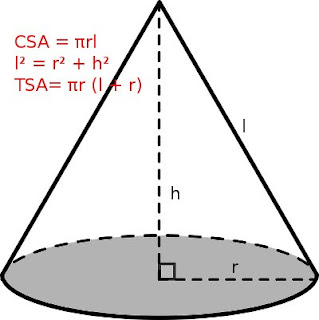## Surface Areas and Volumes

EXERCISE 13.3

Q1: Diameter of the base of a cone is 10.5 cm and its slant height is 10 cm. Find its curved surface area.

Answer: Given r = 10.5/2 = 5.25 cm, l = 10 cm.

Curved surface area (CSA) of the cone = πrl
= (22/7) × 5.25 × 10  = 165 cm² (Answer)

Q2: Find the total surface area of a cone, if its slant height is 21 m and diameter of its base is 24 m.

Answer: Given, l = 21m, r = 24/2 = 12 m
TSA (cone) = πr(l + r)
= (22/7) × 12 (21 + 12)
= 1244.57 m² (Answer)

Q3: Curved surface area of a cone is 308 cm² and its slant height is 14 cm.
Find
(i) radius of the base and
(ii) total surface area of the cone.

(i) Given, l = 14cm, CSA = 308 cm²
CSA = πrl = 308
⇒ r = (308 × 7) / (22 × 2) = 7cm (Answer)

(ii) TSA(cone) = πr (l + r)
= (22/7) × 7 (14 + 7)
= 22 × 21
= 462 cm² (Answer)

Q4: A conical tent is 10 m high and the radius of its base is 24 m. Find
(i) slant height of the tent.
(ii) cost of the canvas required to make the tent, if the cost of 1 m² canvas is Rs 70.

Answer: Given, h = 10 m, r = 24 m
(i) Using Pythagoras theorem, l² = h² + r²
= (10)² + (24)²
= 100 + 576 = 676
⇒ l = √676 = 26m (Answer)

(ii) CSA of conical tent = πrl
= (22/7) × 24 × 26
Cost of 1 m² canvas = ₹ 70
∴ Cost of canvas =  70 × (22/7) × 24 × 26

Q5: What length of tarpaulin 3 m wide will be required to make conical tent of height 8 m and base radius 6 m? Assume that the extra length of material that will be required for Stitching margins and wastage in cutting is approximately 29 cm (use π = 3.14)

Answer: Given h = 6 m, r = 8 m
l² = h² + r²
⇒ l² = 36 + 64 = 100
⇒ l = √100 = 10m

Curved surface area of the tent = πrl
= 3.14 × 6 × 10
∴ required length of tarpaulin = (3.14 × 6 × 10)×⅓ + 0.20m
= 62.8 m + 0.2 m = 63 m (Answer)

Q6: The slant height and base diameter of a conical tomb are 25 m and 14 m respectively. Find the cost of white washing its curved surface at the rate of Rs 210 per 100 m².

Answer: Given, h = 25m, r = 14/2 = 7m

CSA of tomb = πrl
= (22/7) × 7 × 25
= 550 m²
Cost of white washing 100 m² = ₹ 210
∴ Cost of white washing 550 m² = (210/100) × 550 = ₹ 1155 (Answer)

Q7: A joker’s cap is in the form of a right circular cone of base radius 7 cm and height 24 cm. Find the area of the sheet required to make 10 such caps.

Answer: Given, r = 7 cm, h = 24 cm
l² = r² + h²
⇒ l² = 7² + 24² = 49 + 576 = 625
⇒ l = √(625) = 25cm

Total curved surface area of 1 cap = πrl
= (22/7) × 7 × 25
= 550 cm²

Area of sheet required to make 10 such caps = 10 × 550 cm² = 5500 cm² (Answer)

Q8: A bus stop is barricaded from the remaining part of the road, by using 50 hollow cones made of recycled cardboard. Each cone has a base diameter of 40 cm and height 1 m. If the outer side of each of the cones is to be painted and the cost of painting is Rs 12 per m², what will be the cost of painting all these cones? (Use π = 3.14 and take √(1.04) = 1.02)

Answer: Given, r = 40/2 = 20cm = 0.20m, h = 1m
l² = r² + h² = 1² + 0.2² = 1.04
⇒ l = √(1.04) = 1.02

CSA (1 cone) = πrl
CSA (50 cones) = 50πrl
= 50 × 3.14 × 0.2 × 1.02
= 32.028 m²
Cost of painting an area of 1 m² = ₹12
∴ Cost of painting an area of 32.028 m² = Rs 12 × 32.028
= Rs 384.34 (Answer)